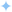Edit on GitHub

# Set up local Postgres

We've provided you several Jupyter notebooks that will help you to shape your data and configure your Postgres instance. You can find these notebooks in the `/tutorials/HRTool/scripts` directory of the repository. We'll walk through each of these notebooks in sequence. It's important to follow the steps in order as they appear, as they are dependent on each other.

## Overview

Using `1_postgres_setup.ipynb`, we'll execute each cell in sequence. Broadly, we're using the `pandas` library to read in the CSV file, convert it to a dataframe, and then write it to a set of tables in Postgres using `pyscopg2` and `sqlalchemy`. We finish by writing a new file called `processed_resume.csv` that we'll use in the next notebook.

The benefit of using these notebooks is that you can check yourself against the output of each cell. Additionally, you can replicate the steps we illustrate for you on your own projects and data. All we're doing is taking a CSV file and getting it into the right shape before then adding it to Postgres.

Below, we walk through the context of what each cell is doing.

## Install dependencies

While there's no cell early on for this, we recommend you install the dependencies for this notebook first:

`pip install pandaspip install psycopg2-binarypip install sqlalchemy`

## Grab the CSV

We're using this dataset from Kaggle. Download it and, inside your repository, add a new set of directories inside the `scripts` folder called, `data/Resume` and place the file in this lowest subdirectory. The filename should be `Resume.csv`.

At this point, you should run the cells in `1_postgres_setup.ipynb` and follow along with the steps below for context.

Here, we're using `pandas` to read in the CSV file. We then use the `head` method to show the first two rows of the dataframe. This is a good way to check that the data is being read in correctly.

`import pandas as pddf = pd.read_csv('data/Resume/Resume.csv')df.head(2)`

## Shape the data

Next, we're using `pandas` to shape the data. We're dropping the `ID`, `Resume_html`, and `Category` columns as we don't need them. We're then renaming the `Resume_str` column to `content` as this is the column that contains the text we'll be vectorizing. We're then adding an `id` column that we'll use as the primary key in our Postgres table. We're also adding a `name` and `email` column that we'll use to populate the `name` and `email` fields in our Postgres table.

Finally, as before, we're using the `head` method to show the first two rows of the dataframe and check ourselves.

`df.drop(columns=['ID','Resume_html', 'Category'], inplace=True)df = df.rename(columns={'Resume_str': 'content'})df['id'] = range(1, len(df) + 1)df['name'] = df['id'].apply(lambda x: f'name_{x}')df['email'] = df['id'].apply(lambda x: f'emailid{x}@mail.com')df.head(2)`

## Create a connection to Postgres

To create a connection to Postgres, we're using the `psycopg2` library. We're using the `connect` method to create a connection to the database after passing in the databases's connection string to the `create_engine` method. This will allow us to execute SQL commands against the database.

`import psycopg2from sqlalchemy import create_engine# Note sqlalchemy has deprecated postgres dialect name so we have to update# env variable HASURA_GRAPHQL_METADATA_DATABASE_URL to postgresql://db = create_engine("postgresql://postgres:postgres@localhost:5432/metadata")conn = db.connect()`

## Create and populate the 'candidate' table

Using the `to_sql` method, we're creating a new table called `candidate` in our Postgres database. We're then populating the table with the `id`, `name`, and `email` columns from our dataframe.

`# Save to Postgres candidates tabledf[['id', 'name', 'email']].to_sql('candidate', conn, index=False, if_exists='append')`

## Create and populate the 'application' table

Similar to the previous step, we're using the `to_sql` method to create a new table called `application` in our Postgres database. We create a new column called `candidate_id` which is a copy of the `id` column, converted to a string.

Then, we create a list of hiring managers which we'll randomly assign to each candidate. Next, we create two new columns: `resume_url`, which is a formatted string containing the `candidate_id`, and `hiring_manager`, which is a randomly selected value from the `hiring_manager_list`.

Finally, we print the number of `null` values in the `candidate_id` column and write all the values to the `application` table.

```# Save to Postgres application tableimport random# df = df.rename(columns={'id': 'candidate_id'})df['candidate_id'] = df['id']df['candidate_id'] = df['candidate_id'].astype(str)
hiring_manager_list = ["Manager1", "Manager2", "Manager3", "Manager4", "Manager5"]df['resume_url'] = df['candidate_id'].apply(lambda x: f'https://www.resume.com/resume_{x}')df['hiring_manager'] = df['candidate_id'].apply(lambda x: random.choice(hiring_manager_list))print(df.candidate_id.isnull().sum())df[['id','candidate_id', 'resume_url', 'hiring_manager']].to_sql('application', conn, index=False, if_exists='append')```

## Write out the processed CSV

Finally, we're writing out the processed CSV file that we'll use in the next notebook.

`df.to_csv("data/Resume/processed_resume.csv", index=False)`
•Build apps and APIs 10x faster
•Built-in authorization and caching
•8x more performant than hand-rolled APIs